Author: Josh Cassidy (August 2013)

This five-part series of articles uses a combination of video and textual descriptions to teach the basics of creating LaTeX graphics using TikZ. These tutorials were first published on the original ShareLateX blog site during August 2013; consequently, today's editor interface (Overleaf) has changed considerably due to the development of ShareLaTeX and the subsequent merger of ShareLaTeX and Overleaf. However, much of the content is still relevant and teaches you some basic LaTeX—skills and expertise that will apply across all platforms.

Additionally, TikZ libraries and methods for positioning nodes have changed over the years. If you're learning TikZ today, it's best to use the positioning library to place your nodes with below=of-style syntax. The positioning library syntax is more flexible and powerful. The older below of=-style syntax used in the video and examples below is officially deprecated but will still work. Additionally, the arrows library is now deprecated in favor of the newer arrows.meta library. Other TikZ commands and libraries used here may have more modern equivalents. Until we produce a new video tutorial, we'll keep the code examples as-is so they correspond with the video content.

In this post we're going to be looking at creating flowcharts in TikZ. To get started we need to load up the tikz package, the shapes.geometric TikZ library and the arrows library.

\usepackage{tikz}
\usetikzlibrary{shapes.geometric, arrows}


### The tikzstyle command

Now before we start the document we need to define the basic components of a flowchart. To do this we use the \tikzstyle command. First, let's define the block we're going to use for start and stop blocks. We'll name it startstop using curly brackets immediately following the command, then we add an equals sign before a set of square brackets. In the square brackets we enter all the formatting information. For this block we'll specify a rectangle with rounded corners. We'll give it a minimum width of 3cm and a minimum height of 1cm. We'll also ensure the text gets centred and we'll set both a draw and a fill colour. In this example we've set the fill colour to a colour that is 30% red mixed with 70% white:

\tikzstyle{startstop} = [rectangle, rounded corners, minimum width=3cm, minimum height=1cm,text centered, draw=black, fill=red!30]


Next we'll specify an input or output box. This time we want the block to be a parallelogram. To achieve this we ask for a trapezium and then alter the angles. The rest is very similar:

\tikzstyle{io} = [trapezium, trapezium left angle=70, trapezium right angle=110, minimum width=3cm, minimum height=1cm, text centered, draw=black, fill=blue!30]


Next we'll add a \tikzstyle for process blocks using a rectangle and a style for decision blocks using a diamond:

\tikzstyle{process} = [rectangle, minimum width=3cm, minimum height=1cm, text centered, draw=black, fill=orange!30]
\tikzstyle{decision} = [diamond, minimum width=3cm, minimum height=1cm, text centered, draw=black, fill=green!30]


Finally we'll define a style for the arrows. For this we set the line thickness to thick, add an arrow head and specify the stealth arrow head:

\tikzstyle{arrow} = [thick,->,>=stealth]


### Nodes

Now we are ready to start building our flowchart. To do the we use the tikzpicture environment. We'll create our flowchart blocks using nodes and the tikzstyles we defined earlier. Nodes are very powerful as we can easily position them, make them draw a shape, heavily format them and give them some text. In square brackets at the end of the begin command we specify a node distance of 2cm. This is so that the nodes we use to build the blocks are automatically spaced 2cm apart from their centres:

\begin{tikzpicture}[node distance=2cm]
<TikZ code>
\end{tikzpicture}


To add a node we use the \node command. We then add a label for the node in parenthesis. This label is how we refer to the node in the rest of the code. Then in square brackets we add the name of the \tikzstyle we want the node to conform to, along with any other formatting options. Then in curly brackets we add the text we want to appear in the block before closing the statement with a semicolon:

\node (start) [startstop] {Start};


If we now compile the code you'll see our start block has appeared as expected: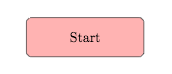Now let's add an input block in below the start block. This time we need to tell the node where to position itself. To do this we enter below of followed by an equals sign and a node label into the square brackets. We could also use above of, right of or left of if we wanted the block to appear somewhere else. We'll tell it to position itself below the start block:

\node (in1) [io, below of=start] {Input};


Now lets add in a process block and a decision block.

\node (pro1) [process, below of=in1] {Process 1};
\node (dec1) [decision, below of=pro1] {Decision 1};


If we compile the code you'll notice that the gap between the green decision block and the orange process block isn't as big as the other gaps: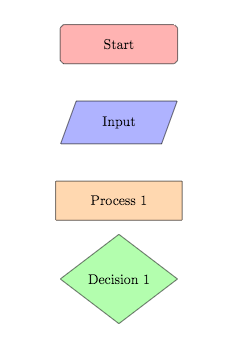This is because the decision block, being a diamond, is taller than the other blocks. Therefore we can manually adjust its position using the yshift variable. If we enter yshift=-0.5cm it will move the decision block vertically down by 0.5cm which should make the gap more regular:

\node (dec1) [decision, below of=pro1, yshift=-0.5cm] {Decision 1};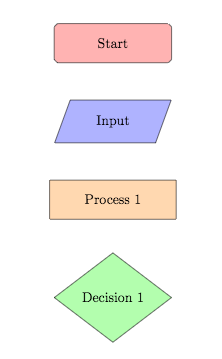Now lets add in two process blocks coming out of the decision block, one below it and one to the right of it. Again, we'll need to alter the positioning using yshift for the block below and xshift for the block to the right. Let's finish off adding the blocks by adding in an output block and a stop block.

\node (pro2a) [process, below of=dec1, yshift=-0.5cm] {Process 2a};
\node (pro2b) [process, right of=dec1, xshift=2cm] {Process 2b};
\node (out1) [io, below of=pro2a] {Output};
\node (stop) [startstop, below of=out1] {Stop};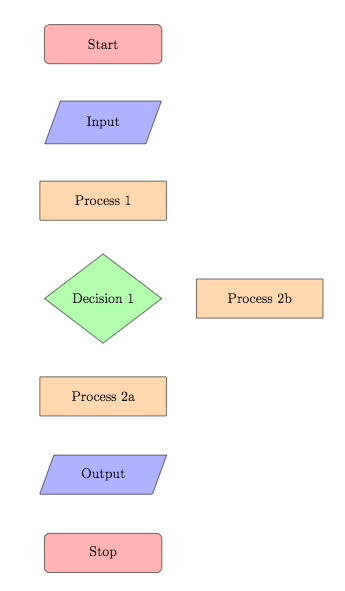### Arrows

To finish off our flowchart we need to add the arrows in. To draw an arrow we use the \draw command and then specify the tikzstyle we prepared for arrows using square brackets. We then enter the label of the node we want the arrow to start from, followed by two dashes and then the label corresponding to the node we want the arrow to terminate at. The labels need to be in parenthesis and we need to make sure we close the statement with a semicolon. Lets add arrows in between the start block and the input block, the input and process 1, process 1 and decision 1, decision 1 and process 2a and between decision 1 and process 2b:

\draw [arrow] (start) -- (in1);
\draw [arrow] (in1) -- (pro1);
\draw [arrow] (pro1) -- (dec1);
\draw [arrow] (dec1) -- (pro2a);
\draw [arrow] (dec1) -- (pro2b);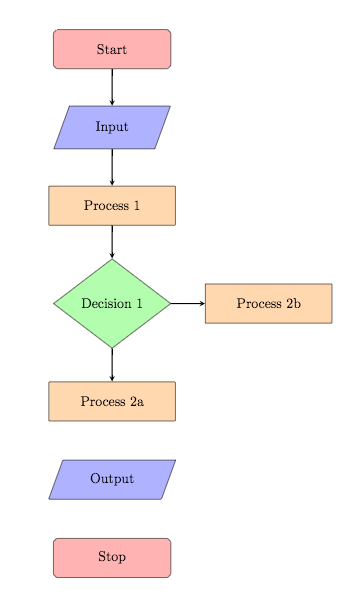As we have arrows coming out of a decision block we need to add some text to these two arrows. To do this we use more nodes, however this time we don't need to use the \node command, we just type the word node in after the two dashes and then the text in curly brackets:

\draw [arrow] (dec1) -- node {yes} (pro2a);
\draw [arrow] (dec1) -- node {no} (pro2b);


If we now compile the code you'll see the text has been added but not in a very helpful place: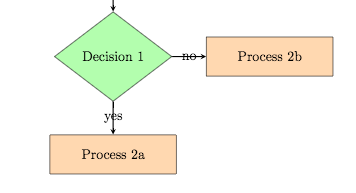To fix this we specify which of the node's anchors TikZ should use to fix the nodes to the lines. To do this we use square brackets immediately after the keyword node and then enter anchor= followed by the anchor. For the yes node we'll use the east anchor and for the no node we'll use the south anchor:

\draw [arrow] (dec1) -- node[anchor=east] {yes} (pro2a);
\draw [arrow] (dec1) -- node[anchor=south] {no} (pro2b);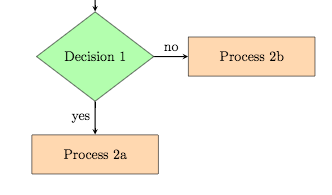Now let's draw the final arrows in:

\draw [arrow] (pro2b) -- (pro1);
\draw [arrow] (pro2a) -- (out1);
\draw [arrow] (out1) -- (stop);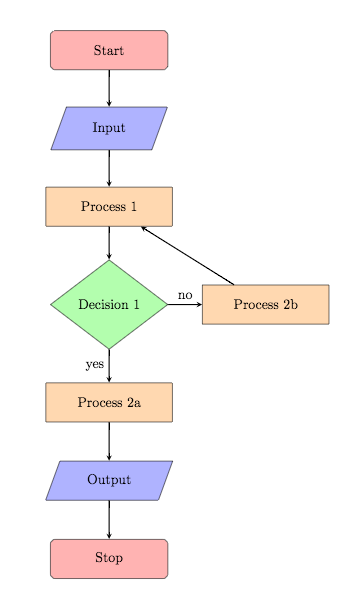You'll also notice that the arrow from process 2b to process 1 is diagonal and therefore doesn't look right. To improve this we can swap the first dash for a bar symbol which will make the arrow go in a vertical direction before going in a horizontal direction:

\draw [arrow] (pro2b) |- (pro1);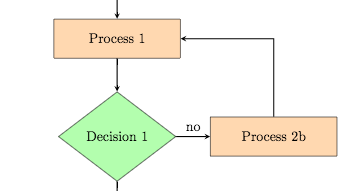### Text width

The final thing we should discuss is the text width. At the moment all our text fits nicely inside our shapes. However, if for example, we add some more text to process 2a, you'll see the shape just extends horizontally until the text fits:

\node (pro2a) [process, below of=dec1, yshift=-0.5cm] {Process 2a text text text text text text text text text text};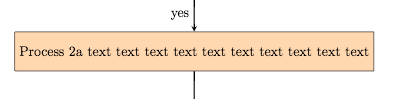This now becomes a bit messy. To improve it we can specify the text width for these nodes by entering text width= followed by a length into our \tikzstyles:

\tikzstyle{process} = [rectangle, minimum width=3cm, minimum height=1cm, text centered, text width=3cm, draw=black, fill=orange!30]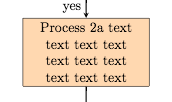This concludes our post on creating flowcharts with TikZ. If you want to play around with the flowchart we created in this post you can access it here. In the next post we'll look at drawing electrical circuits.